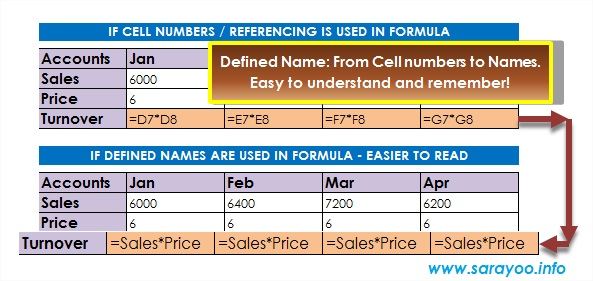# excel defined names

## How to Use Names in Excel Formula and Make it Easier to UnderstandRead: 13,487 Usually we use cell numbers in formulas whenever we work on an Excel spreadsheet. Remembering each cell number and what it contains can be really hard. However, there is an easy way – you can use names in formulas instead of cell numbers. For example: imagine that cell C10 contains Price and cell D10 contains Quantity and you… Read More »How to Use Names in Excel Formula and Make it Easier to Understand